• 深度学习笔记损失函数反向传播公式理解 从机器学习笔记到深度学习笔记直到深度学习的第三章损失函数的反向传播卡了不少时间，发现卡顿的根本原因是对前边的一些细节符号没有准确记忆和串起来，联系上前文一条一条过...
深度学习笔记损失函数反向传播公式过程理解记录
深度学习的第三周课程损失函数的反向传播卡了一点时间，发现卡顿的根本原因是对前边的一些细节符号没有准确记忆，同时过程中一些同一含义的符号发生变化。联系上前文一条一条过终于顺利理解，留些记录免得遗忘。
1.逻辑回归
逻辑回归的公式都比较好理解，深度学习和机器学习笔记都反复提及：2.神经网络中的损失函数计算
以双层神经网络为例，有一个输入层，一个隐藏层和一个输出层。反向传播就是将偏导一层一层写出来，
$dz^{}=\frac{dL}{da^{2}}\cdot \frac{da^{2}}{dz^{2}}$
其中：$L\mathrm{为损失函数定义}:\;L=L(\overset\wedge y^{(i)},y^{(i)})=-y^{(i)}\log\;\overset\wedge y^{(i)}-(1-y^{(i)})\log(1-\;\overset\wedge y^{(i)})\;，\mathrm{此处}\overset\wedge y^{(i)}为a^{\lbrack2\rbrack}\\a^{\lbrack2\rbrack}=\sigma(z^{\lbrack2\rbrack})=\frac1{1+e^{-z^{\lbrack2\rbrack}}}\;;\;\mathrm{其中}\sigma()\mathrm{为激活函数}，\mathrm{教材用的依然是}sigmod\mathrm{函数}$
所以可以得到：
$\frac{da^{\lbrack2\rbrack}}{dz^{\lbrack2\rbrack}}=a^{\lbrack2\rbrack}(1-a^{\lbrack2\rbrack})\;;\;da^{\lbrack2\rbrack}=\frac{dL}{da^{\lbrack2\rbrack}}=-y/a^{\lbrack2\rbrack}+(1-y)/(1-a^{\lbrack2\rbrack})\;\;$
所以：$dz^{}=\frac{dL}{da^{2}}\cdot \frac{da^{2}}{dz^{2}}=a^{}-y$
按两层写一下逻辑回归方程：
$\mathrm{第一层}：z^{\lbrack1\rbrack}=w^{\lbrack1\rbrack}a^{\lbrack0\rbrack}+b^{\lbrack1\rbrack}\;;\;$
$\mathrm{其中}\;a^{\lbrack0\rbrack}=x\;;$
$\mathrm{第一层激活}：a^{\lbrack1\rbrack}=\sigma(z^{\lbrack1\rbrack})\;;$
$\mathrm{第二层}：z^{\lbrack2\rbrack}=w^{\lbrack2\rbrack}a^{\lbrack1\rbrack}+b^{\lbrack2\rbrack}\;;$
$\mathrm{第二层激活}：a^{\lbrack2\rbrack}=\sigma(z^{\lbrack2\rbrack})\;;$
$\mathrm{输出}：\widehat y=a^{\lbrack2\rbrack}\;;$
w在z表达式中，所以对w的求导需要先解L再解z。
$dw=\frac{dL}{dz}\cdot\frac{dz}{dw}=\frac{dL}{dz}\cdot x=dz\cdot x;$
所以由两层逻辑回归方程可以得到：
$dw^{\lbrack2\rbrack}=dz^{\lbrack2\rbrack}\cdot a^{\lbrack1\rbrack}\;;\;dw^{\lbrack1\rbrack}=dz^{\lbrack1\rbrack}\cdot a^{\lbrack0\rbrack}\;,\;\mathrm{其中}a^{\lbrack0\rbrack}=x;$
综上所述，过程1，解第二层需要从输出层往前挨个求偏导。过程2.求第一层也是从输出层挨个往前求偏导，无非就是挨个往前的中间变量可以用过程1的结果做替换，也就是这里面容易跟不上了，一层一层写出来看一遍就会了。
$dz^{\lbrack1\rbrack}=(\frac{dL}{da^{\lbrack2\rbrack}}\cdot\frac{da^{\lbrack2\rbrack}}{dz^{\lbrack2\rbrack}})\cdot\frac{dz^{\lbrack2\rbrack}}{da^{\lbrack1\rbrack}}\cdot\frac{da^{\lbrack1\rbrack}}{dz^{\lbrack1\rbrack}}\;;$
对应的是深度学习笔记中的公式3.4.2：
$dz^{\lbrack1\rbrack}=dz^{\lbrack2\rbrack}\cdot w^{\lbrack2\rbrack T}\cdot\sigma^{\lbrack1\rbrack'}(z^{\lbrack1\rbrack})\;;$
此处在课程笔记中将激活函数用了g()代替了前面部分的函数，含义及表达式一样，或许是为了打字方便，本文中延续机器学习笔记从始至终都用一种符号sigma，便于快速阅读公式。
笔记中公式3.4.1同样的方式展开：
$db^{\lbrack2\rbrack}=\frac{dL}{dz^{\lbrack2\rbrack}}\cdot\frac{dz^{\lbrack2\rbrack}}{db^{\lbrack2\rbrack}}=dz^{\lbrack2\rbrack}\cdot1\;;$
笔记中公式3.44及以后的推导无非是吧代价函数换成了损失函数而已，矩阵的角标及其含义发生变化，过程及形式均一样。


展开全文深度学习 神经网络 经验分享
• Softmax交叉熵损失函数 反向传播公式推导 标签（空格分隔）： Caffe源代码 Softmax交叉熵损失函数： J=−∑i=1Kyiln(zi)zi=exi∑j=1KexjJ=−∑i=1Kyiln⁡(zi)zi=exi∑j=1Kexj\begin{array}{l} J = - \sum\...
Softmax交叉熵损失函数 反向传播公式推导

标签（空格分隔）： Caffe源代码

Softmax交叉熵损失函数：
J=−∑i=1Kyiln(zi)zi=exi∑j=1Kexj$\begin{array}{l}J=-\sum _{i=1}^{K}{y}_{i}\mathrm{ln}\left({z}_{i}\right)\\ {z}_{i}=\frac{{e}^{{x}_{i}}}{\sum _{j=1}^{K}{e}^{{x}_{j}}}\end{array}$\begin{array}{l}
J =  - \sum\limits_{i = 1}^K {{y_i}\ln ({z_i})} \\
{z_i} = \frac{{{e^{{x_i}}}}}{{\sum\limits_{j = 1}^K {{e^{{x_j}}}} }}
\end{array}
现在我们的目的是求∂J∂xk$\frac{\mathrm{\partial }J}{\mathrm{\partial }{x}_{k}}$$\frac{{\partial J}}{{\partial {x_k}}}$

在训练集中，假设 ys=1${y}_{s}=1$$y_{s}=1$，其余yk≠s=0${y}_{k\ne s}=0$$y_{k \ne s} = 0$

我们分为两种情况：
（1）当 k=s$k=s$$k=s$ 时:
∂J∂xk=∂(−ysln(exs∑j=1Kexj))∂xs=−ys×∑j=1Kexjexs×exs×∑j=1Kexj−e2xs(∑j=1Kexj)2=−ys×∑j=1Kexj−exs∑j=1Kexj=−ys(1−zs)$\frac{\mathrm{\partial }J}{\mathrm{\partial }{x}_{k}}=\frac{\mathrm{\partial }\left(-{y}_{s}ln\left(\frac{{e}^{{x}_{s}}}{\sum _{j=1}^{K}{e}^{{x}_{j}}}\right)\right)}{\mathrm{\partial }{x}_{s}}=-{y}_{s}×\frac{\sum _{j=1}^{K}{e}^{{x}_{j}}}{{e}^{{x}_{s}}}×\frac{{e}^{{x}_{s}}×\sum _{j=1}^{K}{e}^{{x}_{j}}-{e}^{2{x}_{s}}}{{\left(\sum _{j=1}^{K}{e}^{{x}_{j}}\right)}^{2}}=-{y}_{s}×\frac{\sum _{j=1}^{K}{e}^{{x}_{j}}-{e}^{{x}_{s}}}{\sum _{j=1}^{K}{e}^{{x}_{j}}}=-{y}_{s}\left(1-{z}_{s}\right)$\frac{{\partial J}}{{\partial {x_k}}} = \frac{{\partial ( - {y_s}ln(\frac{{{e^{{x_s}}}}}{{\sum\limits_{j = 1}^K {{e^{{x_j}}}} }}))}}{{\partial {x_s}}} =  - {y_s} \times \frac{{\sum\limits_{j = 1}^K {{e^{{x_j}}}} }}{{{e^{{x_s}}}}} \times \frac{{{e^{{x_s}}} \times \sum\limits_{j = 1}^K {{e^{{x_j}}}}  - {e^{2{x_s}}}}}{{{{(\sum\limits_{j = 1}^K {{e^{{x_j}}}} )}^2}}} =  - {y_s} \times \frac{{\sum\limits_{j = 1}^K {{e^{{x_j}}}}  - {e^{{x_s}}}}}{{\sum\limits_{j = 1}^K {{e^{{x_j}}}} }} =  - {y_s}(1 - {z_s})

(2)当k≠s$k\ne s$$k \ne s$ 时：
∂J∂xk=∂(−ysln(exs∑j=1Kexj))∂xk=−ys×∑j=1Kexjexs×−exs×exk(∑j=1Kexj)2=−ysexk∑j=1Kexj=−yszk$\frac{\mathrm{\partial }J}{\mathrm{\partial }{x}_{k}}=\frac{\mathrm{\partial }\left(-{y}_{s}ln\left(\frac{{e}^{{x}_{s}}}{\sum _{j=1}^{K}{e}^{{x}_{j}}}\right)\right)}{\mathrm{\partial }{x}_{k}}=-{y}_{s}×\frac{\sum _{j=1}^{K}{e}^{{x}_{j}}}{{e}^{{x}_{s}}}×\frac{-{e}^{{x}_{s}}×{e}^{{x}_{k}}}{{\left(\sum _{j=1}^{K}{e}^{{x}_{j}}\right)}^{2}}=-{y}_{s}\frac{{e}^{{x}_{k}}}{\sum _{j=1}^{K}{e}^{{x}_{j}}}=-{y}_{s}{z}_{k}$\frac{{\partial J}}{{\partial {x_k}}} = \frac{{\partial ( - {y_s}ln(\frac{{{e^{{x_s}}}}}{{\sum\limits_{j = 1}^K {{e^{{x_j}}}} }}))}}{{\partial {x_k}}} =  - {y_s} \times \frac{{\sum\limits_{j = 1}^K {{e^{{x_j}}}} }}{{{e^{{x_s}}}}} \times \frac{{ - {e^{{x_s}}} \times {e^{{x_k}}}}}{{{{(\sum\limits_{j = 1}^K {{e^{{x_j}}}} )}^2}}} =  - {y_s}\frac{{{e^{{x_k}}}}}{{\sum\limits_{j = 1}^K {{e^{{x_j}}}} }} =  - {y_s}{z_k}

总结：
∂J∂xk=zk−yk$\frac{\mathrm{\partial }J}{\mathrm{\partial }{x}_{k}}={z}_{k}-{y}_{k}$\frac{{\partial J}}{{\partial {x_k}}} = z_{k} - y_{k}
展开全文• 文章目录模型交叉熵损失函数及softmax计算误差Python代码 模型 前面得到的Z，然后经过softmax得到输出a，然后根据groud truth y计算损失函数。 交叉熵损失函数及softmax 计算误差 Python代码 def delta_cross_...
文章目录模型交叉熵损失函数及softmax计算误差Python代码
模型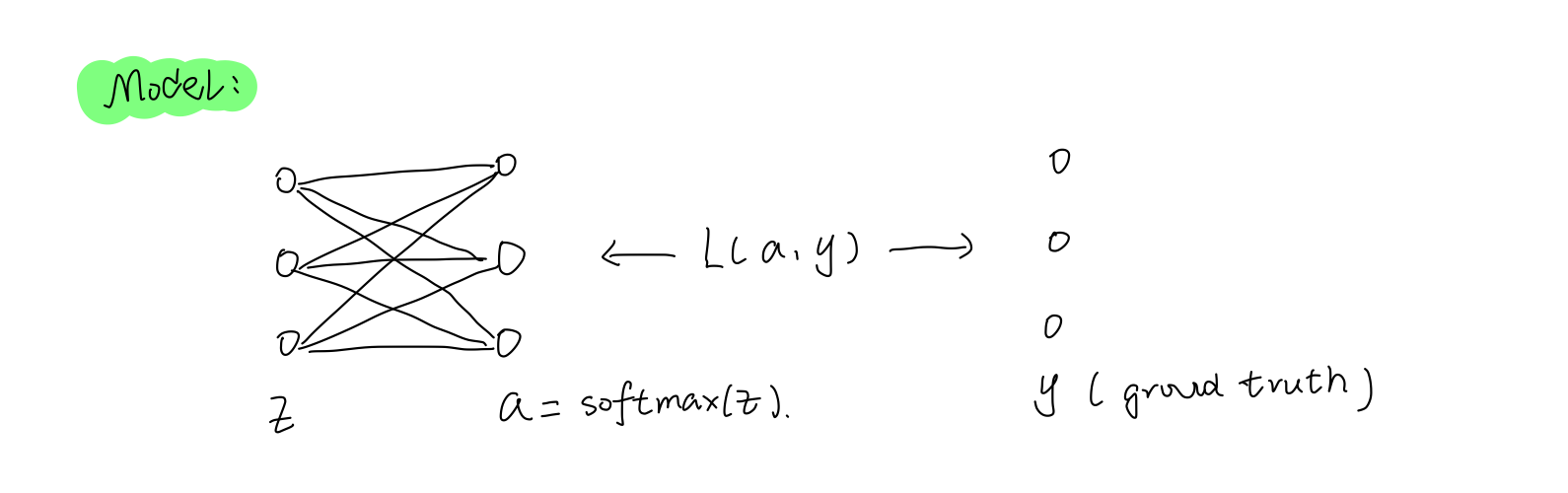前面得到的Z，然后经过softmax得到输出a，然后根据groud truth y计算损失函数。
交叉熵损失函数及softmax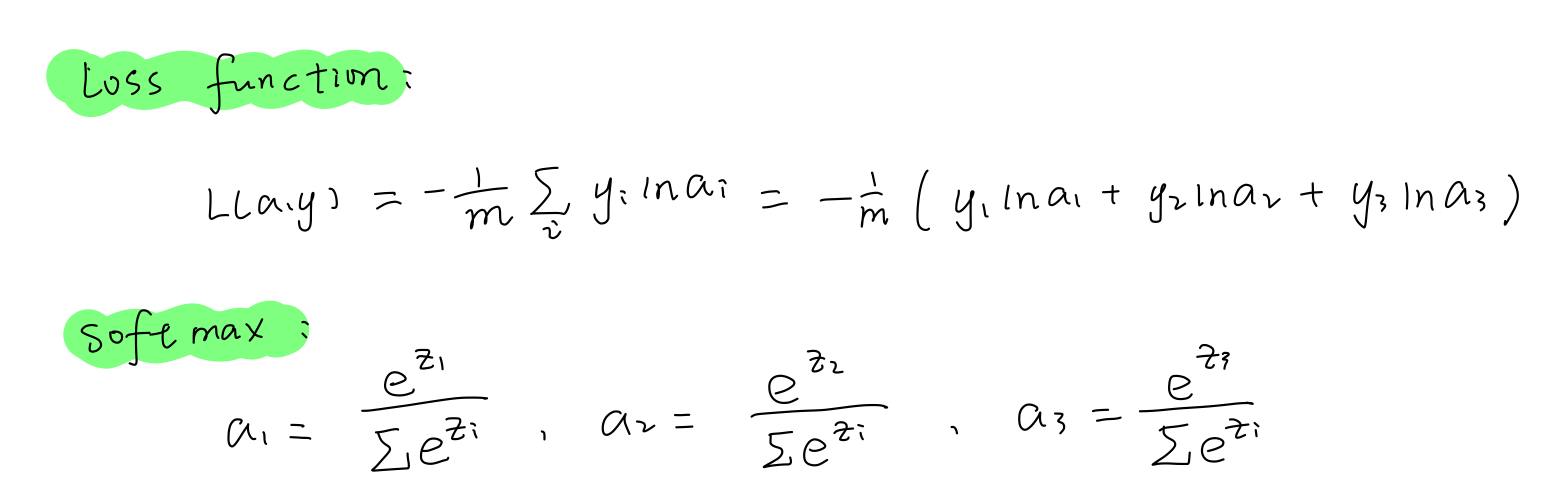计算误差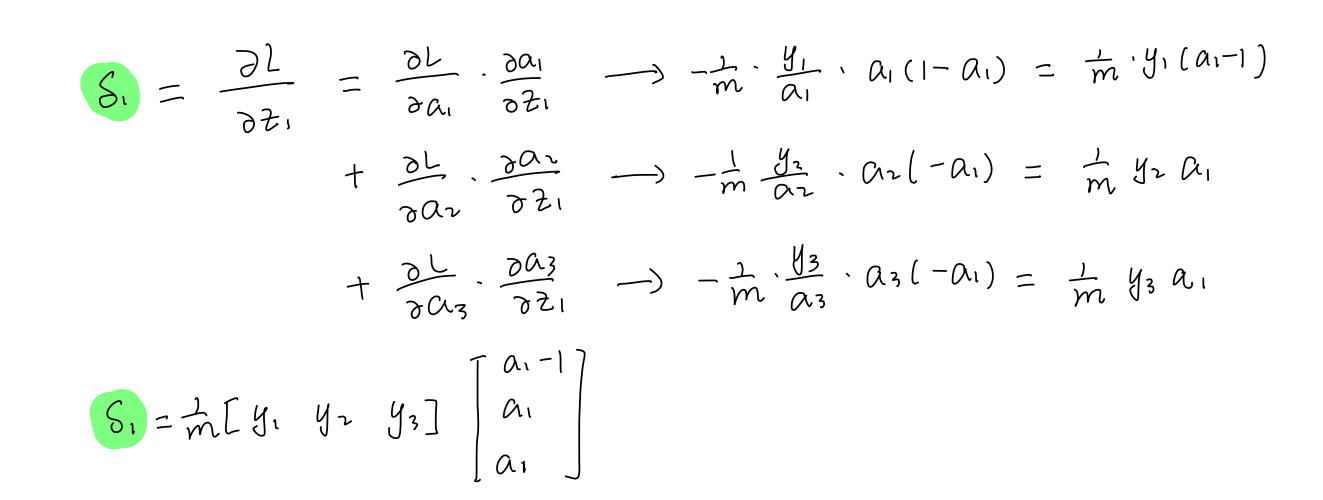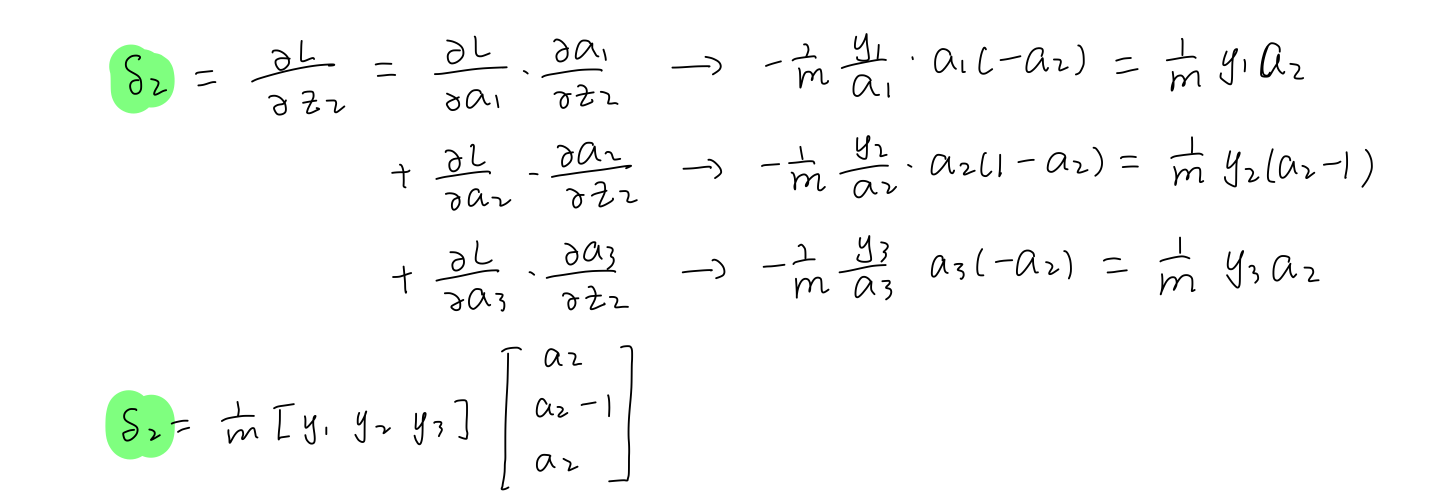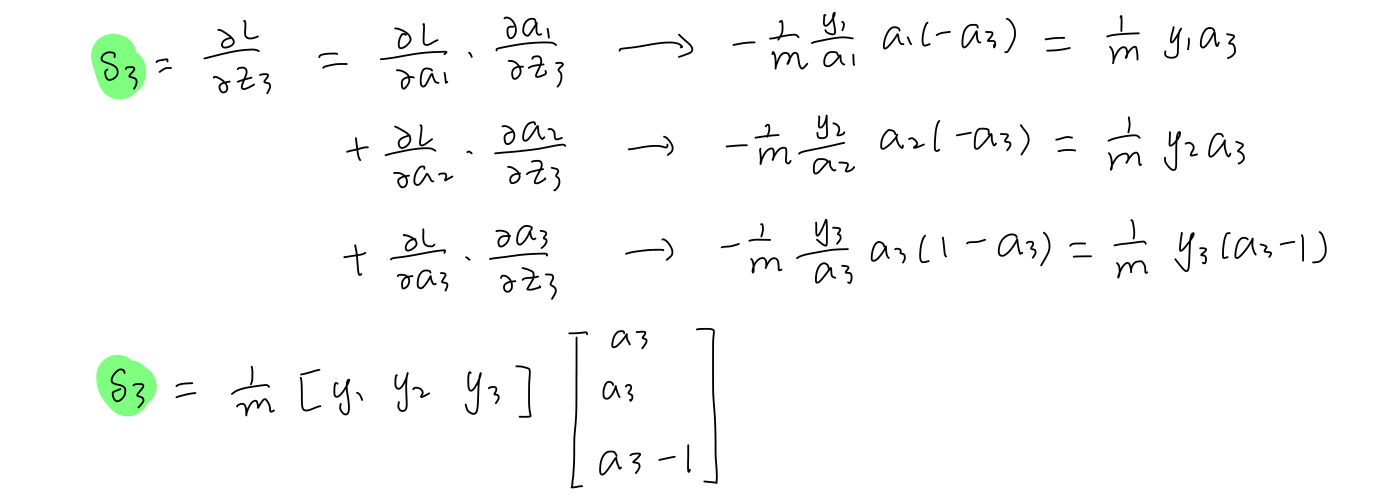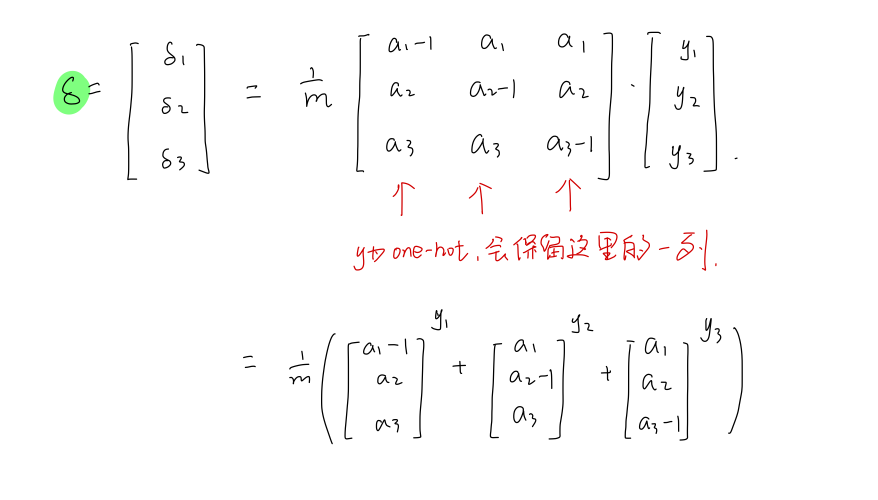Python代码
def delta_cross_entropy(X, y):
'''
X: (num_examples * num_classes) output of fc layer
y: (num_examples * 1) labels
'''
m = y.shape
a = softmax(X)
a[range(m), y] -= 1
delta = a / m
retrun delta

参考：
The Softmax function and its derivative
Softmax和交叉熵的深度解析和Python实现


展开全文softmax
• 当softmax作为中间层的激活函数时，会导致小数相乘，使得效果很不好，所以softmax一般和交叉熵损失函数一起使用，放在最后一层。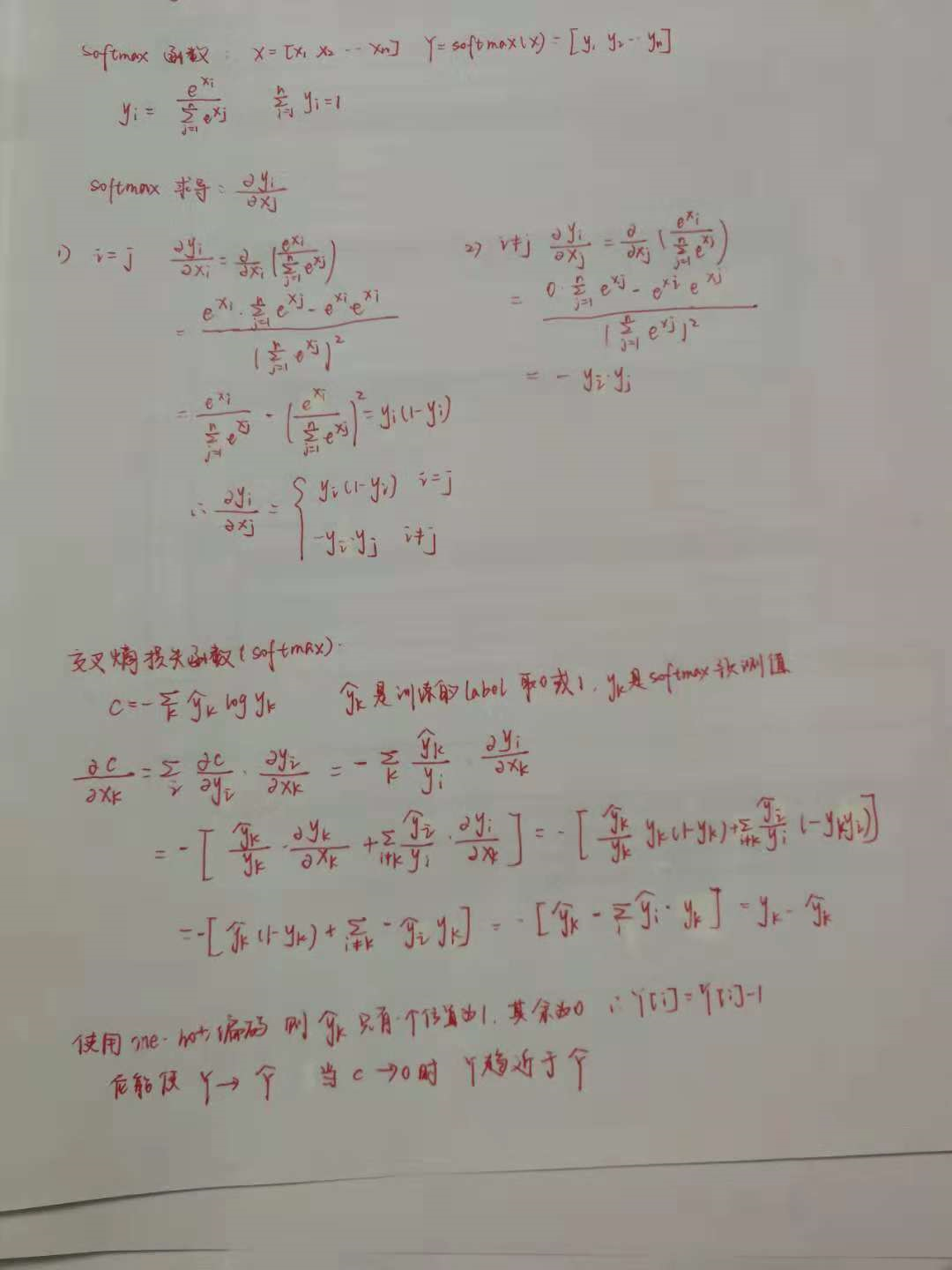当softmax作为中间层的激活函数时，会导致小数相乘，使得效果很不好，所以softmax一般和交叉熵损失函数一起使用，放在最后一层。


展开全文• 目录 一、softmax 函数 二、损失函数 loss function 三、最后的准备工作 ​ 四、具体的推导过程 s...softmax
• 小白提问：pytorch自定义损失函数反向传播也是需要自己写吗？</p>人工智能
• 在二分类问题中用的最多的是...可以看出损失函数基本都是由真实值和预测值两部分组成，正确的损失函数，可以起到让预测值一直逼近真实值的效果，当预测值和真实值相等时，loss值最小。 下面是我以binary_crossentro...
• 在监督学习中，传统的机器学习算法优化过程是采用一个合适的损失函数度量训练样本输出损失，...对DNN的损失函数用梯度下降法进行迭代优化求极小值的过程即为反向传播算法，可以使用多种损失函数和激活函数。 1. 均...
• 常用损失函数 均方差损失 交叉熵损失 依赖知识 a) 了解神经网络的基础知识 b) 熟悉导数的链式法则及常见函数的导数 c) 熟悉常见的优化方法，梯度下降，随机梯度下降等 d) 熟悉矩阵和向量的乘加运算 ...numpy 神经网络
• 关于cross-entropy交叉熵损失函数的详细介绍, 请参考 通过案例详解cross-entropy交叉熵损失函数: https://blog.csdn.net/oBrightLamp/article/details/83962147 1. 定义: E=−∑i=1kyilog(si) E = -\sum_{i = 1...
• Python和PyTorch对比实现cross-entropy交叉熵损失函数反向传播 系列文章索引 : https://blog.csdn.net/oBrightLamp/article/details/85067981 正文 1. 交叉熵函数的定义: 假设在一个多分类问题中, 用 y...深度学习
• 深度学习(一) 损失函数、输出单元、激活函数、反向传播 深度前馈网络 概述 线性模型无论是凸优化还是闭式解都可以高效可靠地拟合，而它的缺陷是拟合能力局限于线性函数里，无法理解特征之间的相互作用。 深度...深度学习
• #设置回归的正确值为两个输入的和加上一个岁计量，加上随机量是为了加入不可预测的噪音，否则不同损失函数的意义就不大了， #因为不同损失函数都会在能完全预测正确的时候最低。一般来说噪音为一个均值为0的小量，...
• 问题背景：多分类问题，输入参数为8组特征...损失函数更新公式： 有几个超参数需要提前设置好，根据自己的问题背景环境。 import numpy as np import datetime import xlrd import matplotlib.pyplot as plt fro..
• RNN网络中，如果某一步骤有输出值，可采用交叉熵定义其损失函数，下面推导了单步传播时，由y_hat（输出值），y（标签）反向传播时的误差传播公式。按照大叔惯例，依然手动，欢迎交流 求得了RNN步传播误差后，就...rnn 深度学习 神经网络
• 本文给出均方差损失函数 MSELoss 的定义并求解其在反向传播中的梯度. 相关 系列文章索引 : https://blog.csdn.net/oBrightLamp/article/details/85067981 正文 均方差损失函数 MSELoss 定义简洁, 梯度求导简单, 应用...
• // 以交叉熵为损失函数的Softmax误差反向传播 推导demo // c++ // 各项的softmax= exp(值) / (所有项的exp(值)之和) // 交叉熵损失= 所有项的 { 期望值 * ln(当前值) } 之和 void SoftmaxCrossEntropyBPDemo()...深度学习
• softmax函数详解及反向传播中的梯度求导[EB/OL]. https://blog.csdn.net/oBrightLamp/article/details/83959185. 有关 cross-entropy 的详细介绍, 请参考 : BrightLamp. 通过案例详解cross-entropy交叉熵损失函数...
• 一、反向传播反向传播：训练模型参数，在所有参数上用梯度下降，使 NN 模型在训练数据上的损失函数最小。 损失函数（loss）：计算得到的预测值 y 与已知答案 y_的差距。 损失函数的计算有很多方法，均方误差 MSE 是...
• # coding: utf-8 import numpy as np # ---------------------------激活函数定义----------------------------... """解决softmax函数的溢出问题，利用c（输入的最大值），softmax函数减去这个最大值保证数据不溢...
• 1凸函数 对于一元函数f(xf(x)，如果对于任意tϵ[0,1]tϵ[0,1]均满足：f(tx1+(1−t)x2)≤tf(x1)+(1−t)f(x2)f(tx1+(1−t)x2)≤tf(x1)+(1−t)f(x2)，则称f(x)f(x)为凸函数(convex function)。 　如果对于任意tϵ(0,1...python...